## Summary and examples

Trigonometric formulas often have to be simplified. This is of importance, for example, when solving equations. In Unit circle and simple formulas we have already seen and used a few common formulas. We will repeat them here again. A few standard formulas are: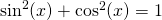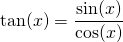Next we have the so-called double-angle formulas: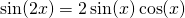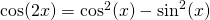The latter formula also occurs in the following forms, depending on the formula to be simplified or the equation to be solved: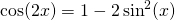Then there are a few formulas that can be derived by means of the unit circle.

Examples are: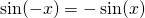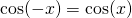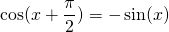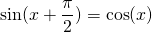Gradually the formulas get more complicated. For example, there are the well-known addition and difference formulas: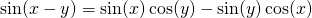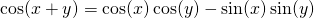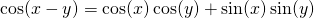Note that the second equation can be easily derived from the first equation. This is also the case with the fourth equation which can be easily derived from the third equation (in the right-hand side substitute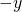for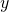and use the following formulas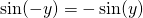and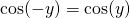.

Finally we have the formulas of Simpson/Mollweide: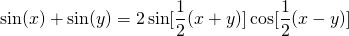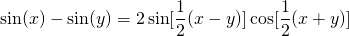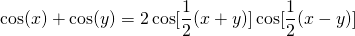These formulas can be derived from the foregoing formulas. Let us take the first formula.

We use the following formulas:By adding both sides of these formulas we get: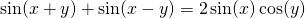If we take: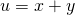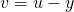we get: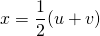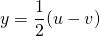and thus: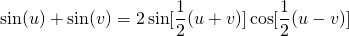Returning to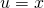and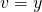we have proven the validity of the first formula of Simpson/Mollweide.

##### Eample 1

Show: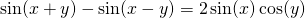We know:By subtracting both sides of these equations we get the formula we have to derive.

##### Example 2

Solve: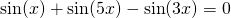For the first two terms we use the formula of: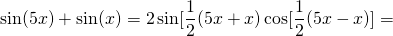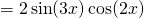and thus we get the equation: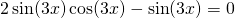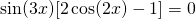This equation yields: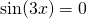or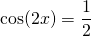The solution of the first equation is: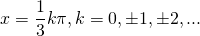The solution of the second equation is: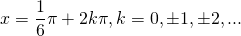or##### Example 3

Solve: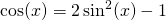We know: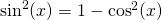and thus we can write: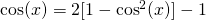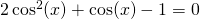Now we call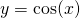and thus we get:This equation can be solved by using the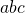-formula and we get: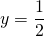of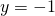Becausewe get: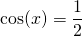with the solution: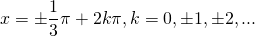or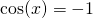with the solution: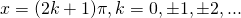0